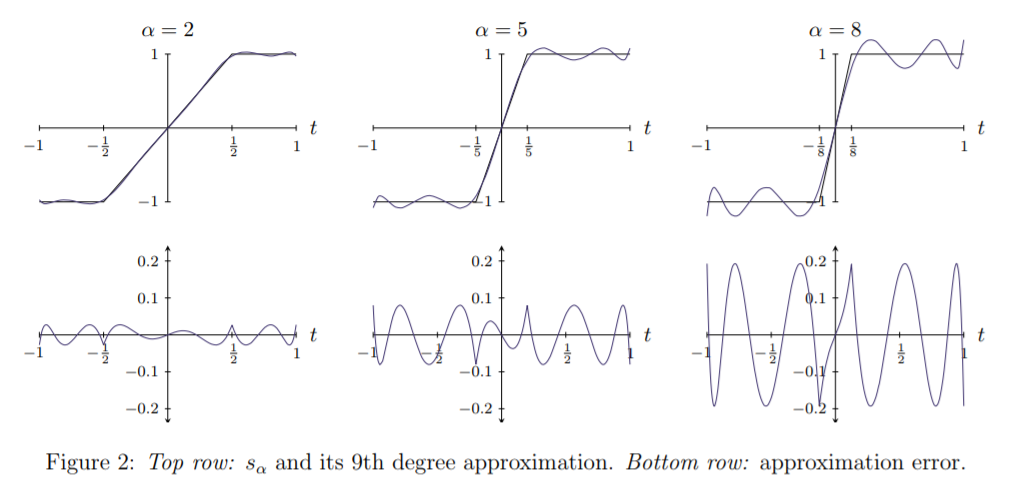# OpenCV—python 自动色彩均衡（ACE）OpenCV/python 同时被 2 个专栏收录83 篇文章 21 订阅

### 一、ACE算法理论

ACE在图像处理方面可以有两种，

1. 对动态范围具有一定的压缩能力；
2. 对亮暗区域的细节有一定的显示能力；
3. 满足上面条件基础上不破坏图像的清晰度。

Rizzi等依据Retinex理论提出了自动颜色均衡算法，该算法考虑了图像中颜色和亮度的空间位置关系，进行局部特性的自适应滤波，实现具有局部和非线性特征的图像亮度与色彩调整和对比度调整，同时满足灰色世界理论假设和白色斑点假设。

##### 1.1 ACE算法
1. 获得空域重构图像
对图像进行色彩/空域调整，完成图像的色差矫正，得到空域重构图像：

R c = ∑ j ∈ s u b s e t , j ≠ p r ( I c ( p ) − I c ( j ) ) d ( p , j ) (1) R_c = \sum_{j\in subset,j \neq p }\frac{r(I_c(p) - I_c(j))}{d(p,j)} \tag{1}

1. 其中 R c ( p ) Rc(p) 是中间结果， I c ( p ) − I c ( j ) I_c(p) - I_c(j) 为2个点的亮度差， d ( p , j ) d(p,j) 表示距离度量函数， r ( ∗ ) r(∗) 为亮度表现函数，需要是奇函数，这一步可以适应局部图像对比度， r ( ∗ ) r(∗) 可以放大较小的差异，并丰富大的差异，根据局部内容扩展或者压缩动态范围。一般的， r ( ∗ ) r(∗) 为:
r ( x ) = { 1 x < − T x / T − T ⩽ x ⩽ T − 1 x > T r(x) = \left\{\begin{matrix} 1 & x<-T\\ x/T & -T \leqslant x \leqslant T\\ -1 & x>T \end{matrix}\right.

2. 对校正后的图像进行动态扩展。ACE算法是对单一色道进行的，对于彩色图片需要对每一个色道分别处理。一种简单的线性扩展可以表示为：
O c ( p ) = r o u n d [ 127.5 + s c R c ( p ) ] (2) O_c(p) = round[127.5+s_cR_c(p)] \tag{2}
其中： s c s_c 斜率为： [ ( m c , 0 ) , ( m c , 255 ) ] [(m_c,0),(m_c,255)] M c = max ⁡ p [ R c ( p ) ] ， m c = min ⁡ p [ R c ( p ) ] M_c = \max_{p}[R_c(p)]，m_c = \min_{p}[R_c(p)]

还可以将其映射到 [ 0 , 255 ] [0,255] 的空间中：
L ( x ) = R ( x ) − min ⁡ R max ⁡ R − min ⁡ R L(x) = \frac{R(x) - \min R}{ \max R - \min R}

a r g min ⁡ I 1 2 ∑ x ( I ( x ) − 1 2 ) 2 − 1 4 M ∑ x ∑ y ≠ x ω ( x , y ) S α ( I ( x ) − I ( y ) ) arg \min_I\frac{1}{2}\sum_{x}(I(x)-\frac{1}{2})^2 -\frac{1}{4M}\sum_x\sum_{y\neq x}\omega(x,y)S\alpha (I(x)-I(y))

S α ′ = s α S_{\alpha }' = s_\alpha
ω = 1 / ∣ ∣ x − y ∣ ∣ \omega =1/||x-y||
M = max ⁡ x R ( x ) M = \max_xR(x)

1. 其他的坡度函数 S α S \alpha ，多项式函数逼近；
2. 除了 1 / ∣ ∣ x − y ∣ ∣ 1/||x-y|| 外的权重函数的选择；
3. 在求和的过程中，y 可以限制在一个小窗口中；
4. L ( x ) L(x) 的一些其他的方法；### 二、快速ACE算法

##### 2.1 原理

ACE的增强效果普遍与retinex好。需要注意的是，ACE中当前像素是与整个图像的其他像素做差分比较，计算复杂度非常非常高，这也是限制它应用的最主要原因。

（1）对一副图像ACE增强后得到输出Y，如果对Y再进行一次ACE增强，输出仍然是Y本身；
（2）对一副图像的ACE增强结果进行尺寸缩放得到Y，对Y进行ACE增强，输出仍然是Y本身。

1. 如何快速的求 I 1 I_1 的ACE增强结果？
其实很简单，对它再次缩放得到 I 2 I_2 ，求 I 2 I_2 的增强结果，依次类推，就是金字塔结构思想。
2. 如何在 Y 1 Y_1 基础上进一步处理得到 Y Y
因为是在整个图像域进行差分比较运算，与近处邻域像素的比较构成了 Y Y 的细节信息，与远处像素的比较构成了 Y Y 的全局背景信息，那么我们合理假设， Y Y Y 1 Y_1 的全局背景信息相同，只更新细节信息即可，也就是，我们需要在 Y 1 Y_1 基础上加上 I I 中邻近像素的差分结果，并减去 Y 1 Y_1 中邻近像素的差分结果就是最终的输出 Y Y

ACE算法的计算公式为：
Y = ∑ g ( I ( x 0 ) − I ( x ) ) ∑ w ( x 0 , x ) Y = \frac{ \sum g(I(x_0)-I(x))}{\sum w(x_0,x)}

x ∈ I x\in I
w w 是权重参数，离中心点像素越远w值越小，可以直接取值欧氏距离。
g ( ) g() 是相对对比度调节参数，非线性的，简单取如下计算方法：
g ( x ) = max ⁡ ( min ⁡ ( a x , 1.0 ) , − 1.0 ) g(x) = \max(\min(ax, 1.0), -1.0)
a是控制参数，值越大，细节增强越明显。计算完后，还要对Y进行一次归一化即可得到最终的增强图像。
##### 2.2 代码演示
import cv2
import numpy as np
import math

def stretchImage(data, s=0.005, bins = 2000):    #线性拉伸，去掉最大最小0.5%的像素值，然后线性拉伸至[0,1]
ht = np.histogram(data, bins);
d = np.cumsum(ht)/float(data.size)
lmin = 0; lmax=bins-1
while lmin<bins:
if d[lmin]>=s:
break
lmin+=1
while lmax>=0:
if d[lmax]<=1-s:
break
lmax-=1
return np.clip((data-ht[lmin])/(ht[lmax]-ht[lmin]), 0,1)

g_para = {}
global g_para
if m is not None:
return m
m = np.zeros((size, size))
if h==0 and w==0:
continue
m /= m.sum()
return m

height,width = I.shape
Z = I[np.ix_(zh, zw)]
res = np.zeros(I.shape)
if para[h][w] == 0:
continue
res += (para[h][w] * np.clip((I-Z[h:h+height, w:w+width])*ratio, -1, 1))
return res

height, width = I.shape[:2]
if min(height, width) <=2:
return np.zeros(I.shape)+0.5
Rs = cv2.resize(I, ((width+1)/2, (height+1)/2))
Rf = zmIceFast(Rs, ratio, radius)             #递归调用
Rf = cv2.resize(Rf, (width, height))
Rs = cv2.resize(Rs, (width, height))

res = np.zeros(I.shape)
for k in range(3):
return res

if __name__ == '__main__':
cv2.imwrite('zmIce.jpg', m)


C++:https://blog.csdn.net/weixin_45709330/article/details/104447450

https://www.cnblogs.com/Imageshop
https://blog.csdn.net/weixin_45709330/article/details/104447336

11-2560208-28622
08-061万+
10-291763
05-14
06-18
12-241516
04-2310万+
08-191057
10-261万+
03-09589
04-113037
09-084089
07-022万+
09-172万+
05-011万+
10-23922点击重新获取扫码支付余额充值